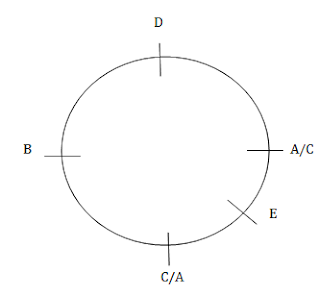# Data Sufficiency Questions from Previous PapersDirections - Each of the following questions below consists of a question and two statements numbered I and II given below it. You have to decide whether the data provided in the statements are sufficient to answer the question.

a) If the data in Statement I alone are sufficient to answer the question, while the data in Statement II alone are not sufficient to answer the question
b) If the data in Statement II alone are sufficient to answer the question,while the data in Statement I alone are not sufficient to answer the question
c) If the data either in Statement I alone or Statement II alone are sufficient to answer the question
d) If the data in both the Statement I and II together are not sufficient to answer the question
e) If the data in both the Statement I and II are together necessary to answer the question

==>> Read Tricks to solve Data Sufficiency questions here

Q1. What is the difference in the age of Paarth and Keshav?
I. Paarth is 20 years older than Mohan
II. Mohan is 2 years younger than Zorawar

Q2. The sum of ages of Manak, Neesha and Opendar is 50 years. What is Neesha's age?
I. Neesha is 10 year older than Manak
II. Opendar is 30 year old

Q3. How many students are there in the class?
I. There are more than 20 but less than that 27 students in the class.
II. There are more than 24 but less than 31 students in the class. The number of students in the class can be divided into groups such that each group contains 5 students.

Q4. Point X is in which direction with respect to Y?
I. Point Z is at equal distance from both point X and point Y.
II. Walking 5 km to the East of point X and taking two consecutive right turns after walking 5 km before each turns lead to point Y.

Q5. Among five friends - Jatin, Kalu, Lucky, Manish and Naresh each of a different height, who is the second tallest?
I. Naresh is taller than Manish and Kalu. Kalu is shorter than Manish.
II. Lucky is taller than Naresh. Jatin is not the tallest.

Q6. How is A related to B?
I. A is the sister - in - law of C, who is the daughter - in - law of B, who is the wife of D
II. B is the mother of A's son only uncle's son

Q7. Among five people - A, B, C, D and E sitting around a circular table facing the center, who is sitting second to the left of D?
I. C is second to the left of A. B and D are immediate neighbors of each other.
II. D is to the immediate left of B. E is not an immediate neighbor of D and B.

Q8. How many sisters does P have?
I. M and T are sisters of K.
D is husband of B who is mother of K and P.

Q9. How is 'call' written in a code language?
I. 'call me back' is written as '531' in that code language.
II. 'you can call me any time' is written as '94163' in that code laguage

Q10. What does '\$' means in a certain code language?
I. '5 \$  # 3' means 'flowers are really good'
II. '7  # 3 5' means 'good flowers are available'.

### Solution

Relation between Paarth and Keshav can not be established with the help of both Statements I and II. So, both the statements are not sufficient to answer the question.

As given in the Question, M + N + O = 50 yr
N = M + 10
So, Now solve the equation
M + N + O = 50
M + (M + 10) + 30 = 50
2 M + 40 = 50
2M = 50 - 40
2M = 10
M = 10/2
M = 5
N = M + 10
N = 5 + 10
N = 15
So, both statements are required to answer the question.

From Statement I
Number of Students in class = 21 or 22 or 23 or 24 or 25 or 26
From Statement II

Number of Students in class = 25 or 26 or 27 or 28 or 29 or 30
Since, number of students can be divided into groups of 5 students, therefore number of students must be multiple of 5.
Number of students in the class = 25 or 30
On combining Statements I and II
Number of students in the class = 25
Hence, the data in both the Statement I and II together are necessary to answer the question.

Statement I is insufficient as no direction is mentioned in it.
From Statement IIHence, X is to the North of Y
So, Statement II alone is sufficient to answer the question.

From Statement I
Naresh > Manish > Kalu
From Statement II
Lucky > Naresh
From both the Statements
Lucky > Naresh > Manish > Kalu
But height of Jatin is not clear.
Thus, second tallest friend can't be determined.
Hence, data neither in Statement I nor in Statement II are sufficient to answer the question.

From Statement IClearly, A is the daughter of B.
From Statement II, A's gender is unknown. Hence, relation can't be established.
So, statement I alone is sufficient to answer the question.

From the statement IFrom Statement IIHence both the statement alone are sufficient
E is sitting second to the left of D.

Both the statements are not sufficient.